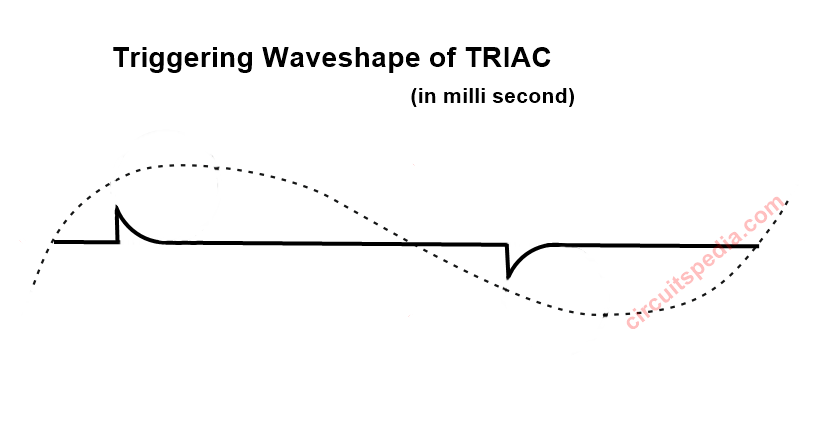# What is DIAC

## What is DIAC

Diac is a device of the thyristor family. DIAC means Diode Alternating current. Diode + Alternating current. This is a type of diode that works in both reverse and forward bias. This diode work on the complete cycle of AC means this work during a positive half-cycle and also during a negative half cycle of the AC waveform. The DIAC is a bidirectional semiconductor switch that can be turned on in both forward and reverse bias polarities of AC signals. This allows current in both forward bias and reverse bias direction.## DIAC Working AND Construction

When the positive supply with MT1 and negative supply with MT2, in this condition Only P1 will work and N1 will not work and P1 and N1 will in Forward bias, N2 and P2 in Reverse bias and P2 and N3 in Forward bias. The Middle junction is in reverse bias. When N2 and P2 are in reverse bias there is very low leakage current flow due to minority carriers. But When the voltage increase then the kinetic energy of electrons of minority charge carriers also increases. And due to increasing voltage, the kinetic energy of electrons of minority carriers are rapidly increased and collides with the electrons of the depletion layer, then created a Breakdown and released a large number of holes and electrons, Now suddenly start the flow of current. As the start of the current conduction across the junction, the Voltage across the junctions decreases. At which voltage the breakdown occurs, that voltage called Breakover voltage VBO.  And After This break over-voltage, DIAC starts conduction.When MT1 is Positive with respect to MT2 then N1 is not working, P1 N2 P2 N3 are working. When MT2 is Positive with respect to MT1 then N3 is not working, Only N1 P1 N2 P2 are working. Diac conducts electrical current only after its break overvoltage. When the voltage across diac increased and get more than Breakover voltage than it starts conduction and the voltage will drop across it. Diac will off and stop the current conduction after the current across diac is less than the Holding current. For both +ve and -ve cycle it works the same but there are small differences in Symmetry, for example, DB3 has a symmetry of 3v means if positive side break over voltage is 32v then the negative side break over voltage is 29v or 35v. There are the possibility to variation in breakover voltage of +ve cycle and -ve cycle of a DIAC.Breakover voltage of DIAC DB3 VBO = 32V (+ – 4v). And for HT60 = 56-70V.

In the given circuit diagram a capacitor and a diac is connected in series with the gate terminal of a Triac. When Switch on, the voltage across diac goes more than break over-voltage and flows a current across it and Triac get a voltage on Gate terminal. And again capacitor gets discharged and then-current conduction goes very less across diac. The current conduction in diac is for a very small moment only. In 220v AC there are Triac gets a Gate current in each cycle and gets triggered for each cycle for a very very small moment (milliseconds). There is a Rise time for a current, Rise time measures the reach current from 10% to 90%. For DB3 DIAC Rise time is 2 microseconds.##Application of DIAC

1. The diac is widely used in triggering of a TRIAC  when used in AC Switches.

2. DIACs are used in the Heat control circuit.

3. In Lamp dimming circuit.

4. In speed control or regulator ckt of fans

5. Also used in starter circuits for Fluorescent Lamps.